# JP4669294B2 - Zoom lens and imaging apparatus having the same - Google Patents

Zoom lens and imaging apparatus having the same Download PDF

## Info

Publication number
JP4669294B2
JP4669294B2 JP2005022561A JP2005022561A JP4669294B2 JP 4669294 B2 JP4669294 B2 JP 4669294B2 JP 2005022561 A JP2005022561 A JP 2005022561A JP 2005022561 A JP2005022561 A JP 2005022561A JP 4669294 B2 JP4669294 B2 JP 4669294B2
Authority
JP
Japan
Prior art keywords
lens
zoom
positive
image
angle
Prior art date
Legal status (The legal status is an assumption and is not a legal conclusion. Google has not performed a legal analysis and makes no representation as to the accuracy of the status listed.)
Active
Application number
JP2005022561A
Other languages
Japanese (ja)
Other versions
JP2006208890A5 (en
JP2006208890A (en
Inventor

Original Assignee
キヤノン株式会社
Priority date (The priority date is an assumption and is not a legal conclusion. Google has not performed a legal analysis and makes no representation as to the accuracy of the date listed.)
Filing date
Publication date
Application filed by キヤノン株式会社 filed Critical キヤノン株式会社
Priority to JP2005022561A priority Critical patent/JP4669294B2/en
Publication of JP2006208890A publication Critical patent/JP2006208890A/en
Publication of JP2006208890A5 publication Critical patent/JP2006208890A5/ja
Application granted granted Critical
Publication of JP4669294B2 publication Critical patent/JP4669294B2/en
Active legal-status Critical Current
Anticipated expiration legal-status Critical

## Links

• 238000003384 imaging method Methods 0.000 title claims description 22
• 239000000463 material Substances 0.000 claims description 12
• 230000004075 alteration Effects 0.000 description 37
• 230000003287 optical Effects 0.000 description 29
• 238000010586 diagrams Methods 0.000 description 23
• 230000014509 gene expression Effects 0.000 description 15
• 201000009310 astigmatism Diseases 0.000 description 6
• 206010010071 Coma Diseases 0.000 description 3
• 239000000470 constituents Substances 0.000 description 3
• 230000000875 corresponding Effects 0.000 description 3
• 210000001747 Pupil Anatomy 0.000 description 2
• 238000006243 chemical reactions Methods 0.000 description 2
• 230000000694 effects Effects 0.000 description 2
• 230000005499 meniscus Effects 0.000 description 2
• 206010073261 Ovarian theca cell tumour Diseases 0.000 description 1
• 208000001644 Thecoma Diseases 0.000 description 1
• 280000407870 Viewfinder companies 0.000 description 1
• 230000003247 decreasing Effects 0.000 description 1
• 238000006073 displacement reactions Methods 0.000 description 1
• 239000011521 glasses Substances 0.000 description 1
• 239000004973 liquid crystal related substances Substances 0.000 description 1
• 239000010950 nickel Substances 0.000 description 1
• 229910052904 quartz Inorganic materials 0.000 description 1
• 239000010453 quartz Substances 0.000 description 1
• 229910001885 silicon dioxide Inorganic materials 0.000 description 1
• 230000001629 suppression Effects 0.000 description 1

## Images

••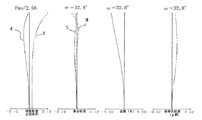•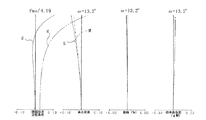•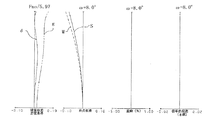•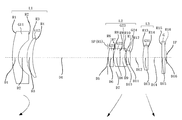•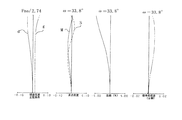•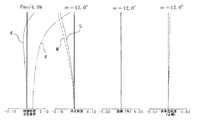•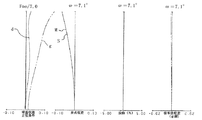•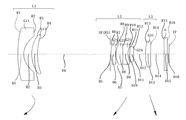•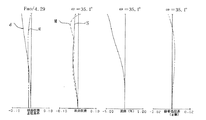•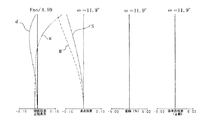•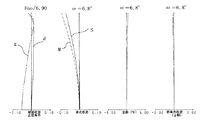•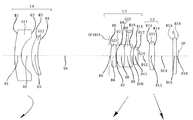•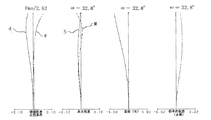•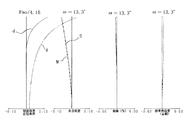•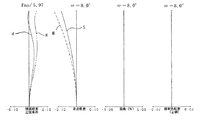•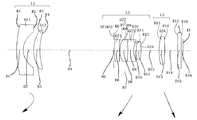•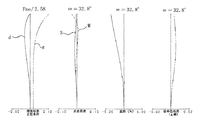•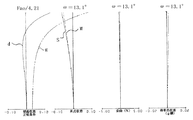•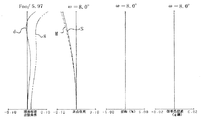•## Classifications

• GPHYSICS
• G02OPTICS
• G02BOPTICAL ELEMENTS, SYSTEMS, OR APPARATUS
• G02B15/00Optical objectives with means for varying the magnification
• G02B15/14Optical objectives with means for varying the magnification by axial movement of one or more lenses or groups of lenses relative to the image plane for continuously varying the equivalent focal length of the objective
• G02B15/16Optical objectives with means for varying the magnification by axial movement of one or more lenses or groups of lenses relative to the image plane for continuously varying the equivalent focal length of the objective with interdependent non-linearly related movements between one lens or lens group, and another lens or lens group
• G02B15/177Optical objectives with means for varying the magnification by axial movement of one or more lenses or groups of lenses relative to the image plane for continuously varying the equivalent focal length of the objective with interdependent non-linearly related movements between one lens or lens group, and another lens or lens group having a negative front lens or group of lenses
• GPHYSICS
• G02OPTICS
• G02BOPTICAL ELEMENTS, SYSTEMS, OR APPARATUS
• G02B15/00Optical objectives with means for varying the magnification
• G02B15/14Optical objectives with means for varying the magnification by axial movement of one or more lenses or groups of lenses relative to the image plane for continuously varying the equivalent focal length of the objective
• G02B15/143Optical objectives with means for varying the magnification by axial movement of one or more lenses or groups of lenses relative to the image plane for continuously varying the equivalent focal length of the objective having three groups only
• G02B15/1435Optical objectives with means for varying the magnification by axial movement of one or more lenses or groups of lenses relative to the image plane for continuously varying the equivalent focal length of the objective having three groups only the first group being negative
• G02B15/143507Optical objectives with means for varying the magnification by axial movement of one or more lenses or groups of lenses relative to the image plane for continuously varying the equivalent focal length of the objective having three groups only the first group being negative arranged -++

## Description

２倍以上のズーム比を有しつつ、レンズ全体をコンパクトな形状にまとめるため、２群ズームレンズの像側に負または正の屈折力の第３レンズ群を配置し、高ズーム比化を図った、所謂３群ズームレンズも提案されている（例えば特許文献１、２）。   In order to bring the entire lens into a compact shape while having a zoom ratio of 2 times or more, a third lens group having a negative or positive refractive power is arranged on the image side of the second group zoom lens to increase the zoom ratio. A so-called three-group zoom lens has also been proposed (for example, Patent Documents 1 and 2).
また、３群ズームレンズとして長いバックフォーカスを有し、かつテレセントリック特性を満足する３群ズームレンズ系も知られている（例えば特許文献３、４）。   A three-group zoom lens system having a long back focus and satisfying telecentric characteristics is also known as a three-group zoom lens (for example, Patent Documents 3 and 4).

In addition, a zoom lens having a high zoom ratio with a zoom ratio of 3 or more is known (for example, Patent Documents 14 to 23).
Japanese Patent Publication No. 7-3507 Japanese Patent Publication No. 6-40170 JP-A 63-135913 Japanese Patent Laid-Open No. 7-261083 JP-A-3-288113 JP-A-9-258103 JP 11-52246 A JP-A-11-174322 JP-A-11-174322 Japanese Patent Application Laid-Open No. 11-194274 Japanese Patent No. 3466385 JP 2002-23053 A JP 2002-196240 A JP-A-4-217219 Japanese Patent Laid-Open No. 10-039214 Japanese Patent No. 10-213745 JP-A-11-119101 JP-A-11-174322 JP 2001-42218 A Japanese Patent No. 2002-3655545 JP 2002-267930 A JP2003-156686A Japanese Patent No. 2895843
３５ｍｍフィルム写真用に設計されている３群ズームレンズは、固体撮像素子を用いる撮像装置に使用するには、バックフォーカスが長すぎ、またテレセントリック特性が良くないため、固体撮像素子を用いる撮像装置にそのまま用いることが難しい。   A three-group zoom lens designed for 35 mm film photography has an excessively long back focus and poor telecentric characteristics for use in an imaging device using a solid-state imaging device. It is difficult to use as it is.

この傾向は、ズームレンズのズーム比が大きくなるほど顕著になる。   This tendency becomes more prominent as the zoom ratio of the zoom lens increases.

１．７＜Δ２Ｘ／√｜ｆ１・ｆ２｜＜２．３
０．５＜Ｄ２３ｗ／ｆｗ＜１．２
１．８８＜ｎ１ａ
なる条件を満足することを特徴としている。
The zoom lens of the present invention includes, in order from the object side to the image side, a first lens unit having a negative refractive power, a second lens unit having a positive refractive power, a third lens unit having positive refractive power, hand during zooming in the zoom lens the distance of each lens group is changed, the wide-angle end from Δ2X the amount of movement of the second lens group during zooming to the telephoto end, the distance between the third lens group and the second lens group at the wide-angle end d23w, the first lens group and the focal length of the second lens group respectively f1, f2, the focal length of the entire system at the wide angle end fw, the average of the refractive index of the material of the lenses constituting the first lens group When the value is n1a ,
1.7 <Δ2X / √ | f1 · f2 | <2.3
0.5 <D23w / fw <1.2
1.88 <n1a
It is characterized by satisfying the following conditions.
この他、本発明のズームレンズは、物体側より像側へ順に、負の屈折力の第１レンズ群、正の屈折力の第２レンズ群、正の屈折力の第３レンズ群からなり、ズーミングに際し各レンズ群の間隔が変化するズームレンズにおいて、前記第１レンズ群は２枚のレンズより成り、広角端から望遠端へのズーミングにおける前記第２レンズ群の移動量をΔ２Ｘ、前記第１レンズ群と前記第２レンズ群の焦点距離を各々ｆ１，ｆ２、前記第１レンズ群を構成するレンズの材料の屈折率の平均値をｎ１ａとするとき、
１．７＜Δ２Ｘ／√｜ｆ１・ｆ２｜＜２．３
１．８８＜ｎ１ａ
なる条件を満足することを特徴としている。
In addition, the zoom lens of the present invention includes, in order from the object side to the image side, a first lens group having a negative refractive power, a second lens group having a positive refractive power, and a third lens group having a positive refractive power. in the zoom lens hand spacing of each lens group is changed upon zooming, the first lens group consists of two lenses, Deruta2X the amount of movement of the second lens group during zooming to the telephoto end from the wide angle end, the first each one lens group is a focal length of the second lens group f1, f2, when an average value of the refractive index of the material of the lenses constituting the first lens group and n1a,
1.7 <Δ2X / √ | f1 · f2 | <2.3
1.88 <n1a
It is characterized by satisfying the following conditions.
この他、本発明のズームレンズは、物体側より像側へ順に、負の屈折力の第１レンズ群、正の屈折力の第２レンズ群、正の屈折力の第３レンズ群からなり、ズーミングに際し各レンズ群の間隔が変化するズームレンズにおいて、広角端から望遠端へのズーミングにおける前記第２レンズ群の移動量をΔ２Ｘ、広角端における前記第２レンズ群と前記第３レンズ群との間隔をＤ２３ｗ、前記第１レンズ群と前記第２レンズ群の焦点距離を各々ｆ１，ｆ２、広角端での全系の焦点距離をｆｗ、前記第２レンズ群を構成する負レンズの材料の屈折率をｎ２ｂとするとき、
１．７＜Δ２Ｘ／√｜ｆ１・ｆ２｜＜２．３
０．５＜Ｄ２３ｗ／ｆｗ＜１．２
１．８５＜ｎ２ｂ
なる条件を満足することを特徴としている。
In addition, the zoom lens of the present invention includes, in order from the object side to the image side, a first lens group having a negative refractive power, a second lens group having a positive refractive power, and a third lens group having a positive refractive power. in the zoom lens hand spacing of each lens group is changed upon zooming, Deruta2X the amount of movement of the second lens group during zooming from the wide-angle end to the telephoto end, and the second lens group at the wide-angle end and the third lens group D23w spacing, the first lens group and the focal length of the second lens group respectively f1, f2, the focal length of the entire system at the wide angle end fw, the material of the negative lens constituting the second lens group When the refractive index is n2b ,
1.7 <Δ2X / √ | f1 · f2 | <2.3
0.5 <D23w / fw <1.2
1.85 <n2b
It is characterized by satisfying the following conditions.
この他、本発明のズームレンズは、物体側より像側へ順に、負の屈折力の第１レンズ群、正の屈折力の第２レンズ群、正の屈折力の第３レンズ群からなり、ズーミングに際し各レンズ群の間隔が変化するズームレンズにおいて、前記第１レンズ群は２枚のレンズより成り、広角端から望遠端へのズーミングにおける前記第２レンズ群の移動量をΔ２Ｘ、前記第１レンズ群と前記第２レンズ群の焦点距離を各々ｆ１，ｆ２、前記第２レンズ群を構成する負レンズの材料の屈折率をｎ２ｂとするとき、
１．７＜Δ２Ｘ／√｜ｆ１・ｆ２｜＜２．３
１．８５＜ｎ２ｂ
なる条件を満足することを特徴としている。
In addition, the zoom lens of the present invention includes, in order from the object side to the image side, a first lens group having a negative refractive power, a second lens group having a positive refractive power, and a third lens group having a positive refractive power. in the zoom lens hand spacing of each lens group is changed upon zooming, the first lens group consists of two lenses, Deruta2X the amount of movement of the second lens group during zooming to the telephoto end from the wide angle end, the first each one lens group is a focal length of the second lens group f1, f2, when the refractive index of the material of the negative lens constituting the second lens group and n2b,
1.7 <Δ2X / √ | f1 · f2 | <2.3
1.85 <n2b
It is characterized by satisfying the following conditions.

Ｇは光学フィルター、フェースプレート、水晶ローパスフィルター、赤外カットフィルター等に相当する光学ブロックである。ＩＰは像面であり、ビデオカメラやデジタルスチルカメラの撮影光学系として使用する際にはＣＣＤセンサやＣＭＯＳセンサ等の固体撮像素子（光電変換素子）の撮像面に相当する感光面が置かれる。   G is an optical block corresponding to an optical filter, a face plate, a quartz low-pass filter, an infrared cut filter, or the like. IP is an image plane, and when used as a photographing optical system of a video camera or a digital still camera, a photosensitive surface corresponding to an imaging surface of a solid-state imaging device (photoelectric conversion device) such as a CCD sensor or a CMOS sensor is placed.

そこで各実施例では、通常の広角レンズと同様、最も物体側のレンズ径の増大が抑えられる負レンズと正レンズのレンズ構成としている。   Therefore, in each embodiment, as with a normal wide-angle lens, a lens configuration of a negative lens and a positive lens that can suppress an increase in the lens diameter closest to the object side is adopted.
そして、負レンズＧ１１の像側のレンズ面をレンズ周辺で負の屈折力が弱くなる非球面形状とすることにより、非点収差と歪曲収差をバランス良く補正すると共に、２枚と言う少ないレンズ枚数で第１レンズ群Ｌ１を構成し、レンズ全体のコンパクト化を図っている。   Then, by making the lens surface on the image side of the negative lens G11 an aspherical shape in which the negative refractive power is weakened around the lens, astigmatism and distortion are corrected in a well-balanced manner and the number of lenses as small as two is reduced. The first lens unit L1 is configured to make the entire lens compact.
また第１レンズ群Ｌ１を構成する各レンズは、軸外主光線の屈折によって生じる軸外収差の発生を抑えるために開口絞りＳＰと光軸が交差する点を中心とする同心球面に近いレンズ形状としている。   Each lens constituting the first lens unit L1 has a lens shape close to a concentric sphere centered on the point where the aperture stop SP intersects the optical axis in order to suppress the occurrence of off-axis aberration caused by refraction of the off-axis principal ray. It is said.

また、正レンズＧ２１は、最も軸上光線の通る高さが高いレンズであり、主に球面収差、コマ収差の補正に関与しているレンズである。そこで各実施例においては、正レンズＧ２１と正レンズＧ２２を配置して、光束を徐々に屈折させることにより球面収差、コマ収差を良好に補正している。   Further, the positive lens G21 is a lens having the highest height of the on-axis light beam, and is a lens mainly involved in correction of spherical aberration and coma aberration. Therefore, in each embodiment, the positive lens G21 and the positive lens G22 are arranged, and the spherical aberration and the coma are corrected well by gradually refracting the light beam.
そして、正レンズＧ２２と接合した負レンズＧ２３の像側の面を、凹形状とするこ
とで、正レンズＧ２１と正レンズＧ２２で発生した収差をキャンセルさせている。
And the aberration which generate | occur | produced with the positive lens G21 and the positive lens G22 is canceled by making the image side surface of the negative lens G23 cemented with the positive lens G22 into a concave shape.

ここで、バックフォーカスをｓｋ’、第３レンズ群Ｌ３の焦点距離をｆ３、第３レンズ群Ｌ３の結像倍率をβ３とすると、
ｓｋ’＝ｆ３（１−β３）
の関係が成り立っている。
Here, when the back focus is sk ′, the focal length of the third lens unit L3 is f3, and the imaging magnification of the third lens unit L3 is β3,
sk ′ = f3 (1-β3)
The relationship is established.

０＜β３＜１．０
である。
However,
0 <β3 <1.0
It is.
ここで、広角端から望遠端へのズーミングに際して第３レンズ群Ｌ３を像側に移動するとバックフォーカスｓｋ’が減少することになり、第３レンズ群Ｌ３の結像倍率β３は望遠側のズーム領域で増大する。すると、結果的に第３レンズ群Ｌ３で変倍を分担することになり、第２レンズ群Ｌ２の移動量が減少し、そのためのスペースが節約できるためにレンズ系の小型化に寄与する。   Here, when the third lens unit L3 is moved to the image side during zooming from the wide-angle end to the telephoto end, the back focus sk ′ is decreased, and the imaging magnification β3 of the third lens unit L3 is a zoom region on the telephoto side. Increase with. Then, as a result, the third lens unit L3 shares the variable magnification, and the amount of movement of the second lens unit L2 is reduced, and the space for this can be saved, contributing to the miniaturization of the lens system.

これは、最も物体側に配置した第１レンズ群Ｌ１をフォーカシングで移動させた場合に生じる、前玉径の増大や、レンズ重量が最も重い第１レンズ群Ｌ１を移動させることによるアクチュエーターの負荷の増大を防ぐことができるからである。また、フォーカスのために第１レンズ群Ｌ１を移動させないことで、第１レンズ群Ｌ１と第２レンズ群Ｌ２とをカム等で単純に連携してズーミング時に移動させることが可能となり、メカ構造の簡素化及び精度向上を達成できる。   This occurs when the first lens unit L1 arranged closest to the object side is moved by focusing. The increase of the front lens diameter and the load of the actuator by moving the first lens unit L1 having the heaviest lens weight are caused. This is because an increase can be prevented. In addition, by not moving the first lens unit L1 for focusing, the first lens unit L1 and the second lens unit L2 can be simply moved together by a cam or the like during zooming, and the mechanical structure is improved. Simplification and improved accuracy can be achieved.
また、第３レンズ群Ｌ３にてフォーカシングを行う場合、広角端から望遠端へのズーミングに際して第３レンズ群Ｌ３を像側に移動させることにより、フォーカシング移動量の大きい望遠端での第３レンズ群Ｌ３の位置を広角端に比して像側に配置することができるため、ズーミング及びフォーカシングで必要となる第３レンズ群Ｌ３の移動量の総和を最小とすることが可能となり、レンズ系のコンパクト化が容易になる。   When focusing is performed by the third lens unit L3, the third lens unit at the telephoto end having a large focusing movement amount is obtained by moving the third lens unit L3 to the image side during zooming from the wide-angle end to the telephoto end. Since the position of L3 can be arranged on the image side compared to the wide-angle end, the total amount of movement of the third lens unit L3 required for zooming and focusing can be minimized, and the lens system is compact. It becomes easy.

０．５＜Ｄ２３ｗ／ｆｗ＜１．２・・・・（２）
３．８＜（β２ｔ・β３）／（β２・β３ｔ）＞５．２・・・・（３）
１．８８＜ｎ１ａ・・・・（４）
１．８５＜ｎ２ｂ・・・・（５）
１．９＜ｆ３／ｆ２＜２．５・・・・（６）
５．２＜ｆ３／ｆｗ＜６．４・・・・（７）
なる条件を満足している。
0.5 < D23w / fw < 1.2 (2)
3.8 <(β2t · β3 w ) / (β2 w · β3t)> 5.2 (3)
1.88 <n1a (4)
1.85 <n2b (5)
1.9 <f3 / f2 <2.5 (6)
5.2 <f3 / fw <6.4 (7)
Is satisfied.

また、条件式（１）の上限値を超えると、第１レンズ群Ｌ１と第２レンズ群Ｌ２のパワーが強くなり、所定のズーム比を確保するための各レンズ群の移動量は減少するため全系のコンパクト化には有利ではあるが、第１レンズ群Ｌ１と第２レンズ群Ｌ２のパワーが強くなることにより非点収差、コマ収差等の諸収差の補正が困難になる。   When the upper limit value of conditional expression (1) is exceeded, the power of the first lens unit L1 and the second lens unit L2 becomes strong, and the amount of movement of each lens unit to ensure a predetermined zoom ratio decreases. Although advantageous for downsizing the entire system, correction of various aberrations such as astigmatism and coma becomes difficult due to the strong power of the first lens unit L1 and the second lens unit L2.

また、条件式（３）の上限を超えると第２レンズ群Ｌ２の変倍分担が大きくなりすぎるため、第２レンズ群Ｌ２を構成するレンズの枚数を増やし、第２レンズ群Ｌ２内での収差の負荷を分散させなければならず、第２レンズ群Ｌ２の全長が長くなり好ましくない。   If the upper limit of conditional expression (3) is exceeded, the variable magnification share of the second lens unit L2 becomes too large. Therefore, the number of lenses constituting the second lens unit L2 is increased, and aberrations in the second lens unit L2 are increased. This is not desirable because the entire length of the second lens unit L2 becomes long.

また、条件式（５）の下限値を超えると、所定のズーム比を得るために第２レンズ群Ｌ２のレンズ構成枚数を増加、あるいは負レンズの肉厚を増大しなければならないため全系のコンパクト化が難しくなる。   If the lower limit of conditional expression (5) is exceeded, the number of lenses constituting the second lens unit L2 must be increased or the thickness of the negative lens must be increased in order to obtain a predetermined zoom ratio. It becomes difficult to make compact.

また、条件式（７）の下限を超えて第３レンズ群Ｌ３のパワーが強くなると、テレセントリック性は良好になるが非点収差が増大し、これを補正するのが困難となる。   Further, when the power of the third lens unit L3 is increased beyond the lower limit of the conditional expression (7), the telecentricity is improved, but the astigmatism is increased, and it is difficult to correct this.

０．６＜Ｄ２３／ｆｗ＜１．１・・・・（２ａ）
３．９＜（β２・β３）／（β２・β３）＜５．１・・・・（３ａ）
１．９０＜ｎ１ａ・・・・（４ａ）
１．９０＜ｎ２ｂ・・・・（５ａ）
２．０＜ｆ３／ｆ２＜２．４・・・・（６ａ）
５．３＜ｆ３／ｆ＜６．３５・・・・（７ａ）
なる条件を満足することである。
0.6 <D23 w /fw<1.1 (2a)
3.9 <(β2 t · β3 w ) / (β2 w · β3 t ) <5.1 (3a)
1.90 <n1a (4a)
1.90 <n2b (5a)
2.0 <f3 / f2 <2.4 (6a)
5.3 <f3 / f w <6.35 ···· (7a)
To satisfy the following conditions.

りに、各レンズ群の間隔が変化するように２つのレンズ群（例えば第１と第２レンズ群、

きる。
In each of the above embodiments, instead of moving the three lens groups during zooming, the two lens groups (for example, the first and second lens groups,
Alternatively, the present invention can also be applied to a zoom type in which the first and third lens groups or the second and third lens groups) are moved.

ンズ群を付加しても本発明で得られる効果には変わりがない。
Further, even if a lens unit having a small refractive power is added to the object side of the first lens unit L1 and / or the image side of the third lens unit L3, the effect obtained by the present invention remains unchanged.

を示し、Ｒｉはレンズ面の曲率半径、Ｄｉは第ｉ面と第（ｉ＋１）面との間の間隔、Ｎｉ
，νｉはそれぞれｄ線を基準とした屈折率、アッベ数を示す。
Next, numerical examples of the present invention will be shown. In each numerical example, i indicates the order of the surfaces from the object side, Ri is the radius of curvature of the lens surface, Di is the distance between the i-th surface and the (i + 1) -th surface, Ni
, Νi respectively indicate the refractive index and Abbe number with respect to the d-line.
また、最も像側の２つの面はフェースプレート等のガラス材である。   The two surfaces closest to the image side are glass materials such as face plates.
また、非球面形状は、光の進行方向を正とし、ｘを光軸方向の面頂点からの変位量、ｈ
を光軸と垂直な方向の光軸からの高さ、Ｒを近軸曲率半径、ｋを円錐定数、Ｂ〜Ｅを非球

ｘ＝（ｈ2／Ｒ）／［１＋｛１−（１＋ｋ）（ｈ／Ｒ）21/2
＋Ｂｈ4＋Ｃｈ6＋Ｄｈ8＋Ｅｈ１０
なる式で表している。
The aspherical shape is such that the light traveling direction is positive, x is the amount of displacement from the surface vertex in the optical axis direction, h
Is the height from the optical axis in the direction perpendicular to the optical axis, R is the paraxial radius of curvature, k is the conic constant, and B to E are aspherical coefficients,
x = (h 2 / R) / [1+ {1− (1 + k) (h / R) 2 } 1/2 ]
+ Bh 4 + Ch 6 + Dh 8 + Eh 10
It is expressed by the following formula.

、ωは半画角を示す。又前述の各条件式と各数値実施例との関係を表１に示す。
“E-0X” means “× 10 −x ”. f represents a focal length, Fno represents an F number, and ω represents a half angle of view. Table 1 shows the relationship between the above-described conditional expressions and numerical examples.

f=4.69〜21.60 Fno=2.56〜5.97 2ω=65.5°〜15.9°

R 1 = 28.278 D 1 = 1.80 N 1 = 1.882997 ν 1 = 40.8
R 2 = 5.123 D 2 = 2.84
R 3 = 9.485 D 3 = 1.75 N 2 = 1.922860 ν 2 = 18.9
R 4 = 16.168 D 4 = 可変
R 5 = 絞り D 5 = 0.40
R 6 = 13.007 D 6 = 1.50 N 3 = 1.693501 ν 3 = 53.2
R 7 = 690.521 D 7 = 0.10
R 8 = 6.185 D 8 = 2.25 N 4 = 1.696797 ν 4 = 55.5
R 9 = -18.336 D 9 = 1.60 N 5 = 1.901355 ν 5 = 31.6
R10 = 5.092 D10 = 0.81
R11 = 23.853 D11 = 1.30 N 6 = 1.719995 ν 6 = 50.2
R12 = -17.457 D12 = 可変
R13 = 18.330 D13 = 1.60 N 7 = 1.487490 ν 7 = 70.2
R14 = -36.938 D14 = 可変
R15 = ∞ D15 = 1.00 N 8 = 1.516330 ν 8 = 64.1
R16 = ∞

D 4 20.33 5.16 1.80
D12 4.02 15.03 26.52
D14 4.33 4.08 3.36

R2 k=-1.57269e+00 B=9.31352e-04 C=-9.32116e-07 D=1.10249e-08
E=3.97985e-10
R8 k=-1.77870e-01 B=5.38565e-05 C=2.46566e-06

f=4.50〜24.35 Fno=2.74〜7.00 2ω=67.7°〜14.1°

R 1 = 28.104 D 1 = 1.80 N 1 = 1.882997 ν1 = 40.8
R 2 = 5.326 D 2 = 2.67
R 3 = 9.520 D 3 = 1.75 N 2 = 1.922860 ν 2 = 18.9
R 4 = 16.301 D 4 = 可変
R 5 = 絞り D 5 = 0.20
R 6 = 10.497 D 6 = 1.50 N 3 = 1.620411 ν 3 = 60.3
R 7 = 91.154 D 7 = 0.10
R 8 = 6.413 D 8 = 2.05 N 4 = 1.788001 ν 4 = 47.4
R 9 = -27.354 D 9 = 1.50 N 5 = 2.003300 ν 5 = 28.3
R10 = 5.137 D10 = 0.50
R11 = 24.649 D11 = 1.20 N 6 = 1.834000 ν 6 = 37.2
R12 = -22.165 D12 = 可変
R13 = 15.118 D13 = 1.60 N 7 = 1.516330 ν 7 = 64.1
R14 = -112.010 D14 = 可変
R15 = ∞ D15 = 1.00 N 8 = 1.516330 ν 8 = 64.1
R16 = ∞

D 4 23.38 5.25 1.80
D12 3.95 16.12 28.69
D14 4.25 3.96 3.25

R2 k=-1.31424e+00 B=6.16883e-04 C=3.16840e-06 D=1.01182e-08
E=-3.58477e-10
R8 k=-2.76663e+00 B=1.25488e-03 C=-1.10959e-05 D=3.02464e-07

f=4.29〜25.30 Fno=2.50〜6.90 2ω=70.2°〜13.6°

R 1 = 33.847 D 1 = 1.80 N 1 = 1.882997 ν 1 = 40.8
R 2 = 5.319 D 2 = 2.49
R 3 = 9.526 D 3 = 1.75 N 2 = 1.922860 ν 2 = 18.9
R 4 = 16.478 D 4 = 可変
R 5 = 絞り D 5 = 0.20
R 6 = 10.394 D 6 = 1.45 N 3 = 1.639999 ν 3 = 60.1
R 7 = 62.083 D 7 = 0.10
R 8 = 6.334 D 8 = 2.10 N 4 = 1.772499 ν 4 = 49.6
R 9 = -51.827 D 9 = 1.55 N 5 = 2.003300 ν 5 = 28.3
R10 = 5.096 D10 = 0.55
R11 = 22.577 D11 = 1.20 N 6 = 1.834000 ν 6 = 37.2
R12 = -21.511 D12 = 可変
R13 = 12.440 D13 = 1.60 N 7 = 1.516330 ν 7 = 64.1
R14 = 110.419 D14 = 可変
R15 = ∞ D15 = 1.00 N 8 = 1.516330 ν 8 = 64.1
R16 = ∞

D 4 22.31 4.94 1.78
D12 3.54 16.87 30.62
D14 4.16 3.50 2.42

R1 k=1.66792e+01 B=3.29890e-05 C=-3.75031e-06 D=7.54949e-08
E=-8.64945e-10
R2 k=-1.28756e+00 B=6.38450e-04 C=5.28748e-06 D=-1.07260e-07
E=7.71211e-10
R8 k=-3.00310e+00 B=1.38557e-03 C=-1.77869e-05 D=5.11304e-07

f=4.69〜21.60 Fno=2.52〜5.97 2ω=65.5°〜15.9°

R 1 = 27.781 D 1 = 1.80 N 1 = 1.882997 ν 1 = 40.8
R 2 = 5.112 D 2 = 2.87
R 3 = 9.459 D 3 = 1.75 N 2 = 1.922860 ν 2 = 18.9
R 4 = 16.081 D 4 = 可変
R 5 = 絞り D 5 = 0.40
R 6 = 12.693 D 6 = 1.50 N 3 = 1.693501 ν 3 = 53.2
R 7 = 748.560 D 7 = 0.10
R 8 = 6.188 D 8 = 2.25 N 4 = 1.696797 ν 4 = 55.5
R 9 = -17.856 D 9 = 1.60 N 5 = 1.901355 ν 5 = 31.6
R10 = 5.062 D10 = 0.90
R11 = 24.749 D11 = 1.30 N 6 = 1.719995 ν 6 = 50.2
R12 = -17.554 D12 = 可変
R13 = 20.052 D13 = 1.60 N 7 = 1.487490 ν 7 = 70.2
R14 = -32.668 D14 = 可変
R15 = ∞ D15 = 1.00 N 8 = 1.516330 ν 8 = 64.1
R16 = ∞

D 4 19.84 5.11 1.80
D12 3.00 14.28 26.27
D14 4.99 4.58 3.48

R2 k=-1.55604e+00 B=9.15097e-04 C=-5.65375e-08 D=7.02344e-09
E=2.63734e-10
R8 k=-2.86351e-01 B=1.03856e-04 C=4.26746e-06

f=4.69〜21.60 Fno=2.58〜5.97 2ω=65.5°〜15.9°

R 1 = 28.388 D 1 = 1.80 N 1 = 1.882997 ν 1 = 40.8
R 2 = 5.087 D 2 = 2.90
R 3 = 9.529 D 3 = 1.75 N 2 = 1.922860 ν 2 = 18.9
R 4 = 16.168 D 4 = 可変
R 5 = 絞り D 5 = 0.10
R 6 = 13.199 D 6 = 1.50 N 3 = 1.693501 ν 3 = 53.2
R 7 = 554.461 D 7 = 0.10
R 8 = 6.184 D 8 = 2.25 N 4 = 1.696797 ν 4 = 55.5
R 9 = -18.358 D 9 = 1.60 N 5 = 1.901355 ν 5 = 31.6
R10 = 5.115 D10 = 0.81
R11 = 22.733 D11 = 1.30 N 6 = 1.719995 ν 6 = 50.2
R12 = -16.955 D12 = 可変
R13 = 16.884 D13 = 1.60 N 7 = 1.487490 ν 7 = 70.2
R14 = -49.680 D14 = 可変
R15 = ∞ D15 = 1.00 N 8 = 1.516330 ν 8 = 64.1
R16 = ∞

D 4 20.79 5.40 1.96
D12 5.06 15.84 26.79
D14 3.58 3.27 2.80

R2 k=-1.49618e+00 B=8.75196e-04 C=-3.59972e-07 D=1.41022e-08
E=3.46972e-10
R8 k=-4.33552e-01 B=1.86343e-04 C=5.39556e-06

Numerical example 1

f = 4.69-21.60 Fno = 2.56-5.97 2ω = 65.5 ° -15.9 °

R 1 = 28.278 D 1 = 1.80 N 1 = 1.882997 ν 1 = 40.8
R 2 = 5.123 D 2 = 2.84
R 3 = 9.485 D 3 = 1.75 N 2 = 1.922860 ν 2 = 18.9
R 4 = 16.168 D 4 = Variable
R 5 = Aperture D 5 = 0.40
R 6 = 13.007 D 6 = 1.50 N 3 = 1.693501 ν 3 = 53.2
R 7 = 690.521 D 7 = 0.10
R 8 = 6.185 D 8 = 2.25 N 4 = 1.696797 ν 4 = 55.5
R 9 = -18.336 D 9 = 1.60 N 5 = 1.901355 ν 5 = 31.6
R10 = 5.092 D10 = 0.81
R11 = 23.853 D11 = 1.30 N 6 = 1.719995 ν 6 = 50.2
R12 = -17.457 D12 = variable
R13 = 18.330 D13 = 1.60 N 7 = 1.487490 ν 7 = 70.2
R14 = -36.938 D14 = variable
R15 = ∞ D15 = 1.00 N 8 = 1.516330 ν 8 = 64.1
R16 = ∞

Focal length 4.69 12.90 21.60
Variable interval
D 4 20.33 5.16 1.80
D12 4.02 15.03 26.52
D14 4.33 4.08 3.36

Aspheric coefficient
R2 k = -1.57269e + 00 B = 9.31352e-04 C = -9.32116e-07 D = 1.10249e-08
E = 3.97985e-10
R8 k = -1.77870e-01 B = 5.38565e-05 C = 2.46566e-06

Numerical example 2

f = 4.50〜24.35 Fno = 2.74〜7.00 2ω = 67.7 ° 〜14.1 °

R 1 = 28.104 D 1 = 1.80 N 1 = 1.882997 ν1 = 40.8
R 2 = 5.326 D 2 = 2.67
R 3 = 9.520 D 3 = 1.75 N 2 = 1.922860 ν 2 = 18.9
R 4 = 16.301 D 4 = Variable
R 5 = Aperture D 5 = 0.20
R 6 = 10.497 D 6 = 1.50 N 3 = 1.620411 ν 3 = 60.3
R 7 = 91.154 D 7 = 0.10
R 8 = 6.413 D 8 = 2.05 N 4 = 1.788001 ν 4 = 47.4
R 9 = -27.354 D 9 = 1.50 N 5 = 2.003300 ν 5 = 28.3
R10 = 5.137 D10 = 0.50
R11 = 24.649 D11 = 1.20 N 6 = 1.834000 ν 6 = 37.2
R12 = -22.165 D12 = variable
R13 = 15.118 D13 = 1.60 N 7 = 1.516330 ν 7 = 64.1
R14 = -112.010 D14 = variable
R15 = ∞ D15 = 1.00 N 8 = 1.516330 ν 8 = 64.1
R16 = ∞

Focal length 4.50 14.14 24.35
Variable interval
D 4 23.38 5.25 1.80
D12 3.95 16.12 28.69
D14 4.25 3.96 3.25

Aspheric coefficient
R2 k = -1.31424e + 00 B = 6.16883e-04 C = 3.16840e-06 D = 1.01182e-08
E = -3.58477e-10
R8 k = -2.76663e + 00 B = 1.25488e-03 C = -1.10959e-05 D = 3.02464e-07

Numerical Example 3

f = 4.29-25.30 Fno = 2.50-6.90 2ω = 70.2 ° -13.6 °

R 1 = 33.847 D 1 = 1.80 N 1 = 1.882997 ν 1 = 40.8
R 2 = 5.319 D 2 = 2.49
R 3 = 9.526 D 3 = 1.75 N 2 = 1.922860 ν 2 = 18.9
R 4 = 16.478 D 4 = Variable
R 5 = Aperture D 5 = 0.20
R 6 = 10.394 D 6 = 1.45 N 3 = 1.639999 ν 3 = 60.1
R 7 = 62.083 D 7 = 0.10
R 8 = 6.334 D 8 = 2.10 N 4 = 1.772499 ν 4 = 49.6
R 9 = -51.827 D 9 = 1.55 N 5 = 2.003300 ν 5 = 28.3
R10 = 5.096 D10 = 0.55
R11 = 22.577 D11 = 1.20 N 6 = 1.834000 ν 6 = 37.2
R12 = -21.511 D12 = variable
R13 = 12.440 D13 = 1.60 N 7 = 1.516330 ν 7 = 64.1
R14 = 110.419 D14 = variable
R15 = ∞ D15 = 1.00 N 8 = 1.516330 ν 8 = 64.1
R16 = ∞

Focal length 4.29 14.31 25.30
Variable interval
D 4 22.31 4.94 1.78
D12 3.54 16.87 30.62
D14 4.16 3.50 2.42

Aspheric coefficient
R1 k = 1.66792e + 01 B = 3.29890e-05 C = -3.75031e-06 D = 7.54949e-08
E = -8.64945e-10
R2 k = -1.28756e + 00 B = 6.38450e-04 C = 5.28748e-06 D = -1.07260e-07
E = 7.71211e-10
R8 k = -3.00310e + 00 B = 1.38557e-03 C = -1.77869e-05 D = 5.11304e-07

Numerical Example 4

f = 4.69〜21.60 Fno = 2.52〜5.97 2ω = 65.5 ° 〜15.9 °

R 1 = 27.781 D 1 = 1.80 N 1 = 1.882997 ν 1 = 40.8
R 2 = 5.112 D 2 = 2.87
R 3 = 9.459 D 3 = 1.75 N 2 = 1.922860 ν 2 = 18.9
R 4 = 16.081 D 4 = variable
R 5 = Aperture D 5 = 0.40
R 6 = 12.693 D 6 = 1.50 N 3 = 1.693501 ν 3 = 53.2
R 7 = 748.560 D 7 = 0.10
R 8 = 6.188 D 8 = 2.25 N 4 = 1.696797 ν 4 = 55.5
R 9 = -17.856 D 9 = 1.60 N 5 = 1.901355 ν 5 = 31.6
R10 = 5.062 D10 = 0.90
R11 = 24.749 D11 = 1.30 N 6 = 1.719995 ν 6 = 50.2
R12 = -17.554 D12 = variable
R13 = 20.052 D13 = 1.60 N 7 = 1.487490 ν 7 = 70.2
R14 = -32.668 D14 = variable
R15 = ∞ D15 = 1.00 N 8 = 1.516330 ν 8 = 64.1
R16 = ∞

Focal length 4.69 17.55 21.60
Variable interval
D 4 19.84 5.11 1.80
D12 3.00 14.28 26.27
D14 4.99 4.58 3.48

Aspheric coefficient
R2 k = -1.55604e + 00 B = 9.15097e-04 C = -5.65375e-08 D = 7.02344e-09
E = 2.63734e-10
R8 k = -2.86351e-01 B = 1.03856e-04 C = 4.26746e-06

Numerical Example 5

f = 4.69-21.60 Fno = 2.58-5.97 2ω = 65.5 ° -15.9 °

R 1 = 28.388 D 1 = 1.80 N 1 = 1.882997 ν 1 = 40.8
R 2 = 5.087 D 2 = 2.90
R 3 = 9.529 D 3 = 1.75 N 2 = 1.922860 ν 2 = 18.9
R 4 = 16.168 D 4 = Variable
R 5 = Aperture D 5 = 0.10
R 6 = 13.199 D 6 = 1.50 N 3 = 1.693501 ν 3 = 53.2
R 7 = 554.461 D 7 = 0.10
R 8 = 6.184 D 8 = 2.25 N 4 = 1.696797 ν 4 = 55.5
R 9 = -18.358 D 9 = 1.60 N 5 = 1.901355 ν 5 = 31.6
R10 = 5.115 D10 = 0.81
R11 = 22.733 D11 = 1.30 N 6 = 1.719995 ν 6 = 50.2
R12 = -16.955 D12 = variable
R13 = 16.884 D13 = 1.60 N 7 = 1.487490 ν 7 = 70.2
R14 = -49.680 D14 = variable
R15 = ∞ D15 = 1.00 N 8 = 1.516330 ν 8 = 64.1
R16 = ∞

Focal length 4.69 12.98 21.60
Variable interval
D 4 20.79 5.40 1.96
D12 5.06 15.84 26.79
D14 3.58 3.27 2.80

Aspheric coefficient
R2 k = -1.49618e + 00 B = 8.75196e-04 C = -3.59972e-07 D = 1.41022e-08
E = 3.46972e-10
R8 k = -4.33552e-01 B = 1.86343e-04 C = 5.39556e-06

このように本発明のズームレンズをデジタルスチルカメラ等の撮像装置に適用することにより、小型で高い光学性能を有する撮像装置を実現している。   Thus, by applying the zoom lens of the present invention to an image pickup apparatus such as a digital still camera, a small image pickup apparatus having high optical performance is realized.

Ｌ１ 第１レンズ群
Ｌ２ 第２レンズ群
Ｌ３ 第３レンズ群
ＳＰ 絞り
ＩＰ 像面
Ｇ ガラスブロック
ｄ ｄ線
ｇ ｇ線
ΔＳ サジタル像面
ΔＭ メリディオナル像面
L1 First lens group L2 Second lens group L3 Third lens group SP Aperture IP Image plane G Glass block d d line g g line ΔS Sagittal image plane ΔM Meridional image plane

## Claims (17)

1. 物体側より像側へ順に、負の屈折力の第１レンズ群、正の屈折力の第２レンズ群、正の屈折力の第３レンズ群からなり、ズーミングに際し各レンズ群の間隔が変化するズームレンズにおいて、広角端から望遠端へのズーミングにおける前記第２レンズ群の移動量をΔ２Ｘ、広角端における前記第２レンズ群と前記第３レンズ群との間隔をＤ２３ｗ、前記第１レンズ群と前記第２レンズ群の焦点距離を各々ｆ１，ｆ２、広角端での全系の焦点距離をｆｗ、前記第１レンズ群を構成するレンズの材料の屈折率の平均値をｎ１ａとするとき、
１．７＜Δ２Ｘ／√｜ｆ１・ｆ２｜＜２．３
０．５＜Ｄ２３ｗ／ｆｗ＜１．２
１．８８＜ｎ１ａ
なる条件を満足することを特徴とするズームレンズ。
In order from the object side to the image side, a first lens unit having a negative refractive power, a second lens unit having a positive refractive power and a positive third lens group refractive power, hand spacing of each lens group changes upon zooming in the zoom lens, Deruta2X the movement amount of the second lens group in zooming from the wide-angle end to the telephoto end, d23w the distance between the third lens group and the second lens group at the wide-angle end, the first lens group when the focal length of each of the f1 of the second lens group, f2, the focal length of the entire system at the wide angle end fw, the average value of the refractive index of the material of the lenses constituting the first lens group and n1a and,
1.7 <Δ2X / √ | f1 · f2 | <2.3
0.5 <D23w / fw <1.2
1.88 <n1a
A zoom lens that satisfies the following conditions:
2. 物体側より像側へ順に、負の屈折力の第１レンズ群、正の屈折力の第２レンズ群、正の屈折力の第３レンズ群からなり、ズーミングに際し各レンズ群の間隔が変化するズームレンズにおいて、前記第１レンズ群は２枚のレンズより成り、広角端から望遠端へのズーミングにおける前記第２レンズ群の移動量をΔ２Ｘ、前記第１レンズ群と前記第２レンズ群の焦点距離を各々ｆ１，ｆ２、前記第１レンズ群を構成するレンズの材料の屈折率の平均値をｎ１ａとするとき、
１．７＜Δ２Ｘ／√｜ｆ１・ｆ２｜＜２．３
１．８８＜ｎ１ａ
なる条件を満足することを特徴とするズームレンズ。
In order from the object side to the image side, a first lens unit having a negative refractive power, a second lens unit having a positive refractive power and a positive third lens group refractive power, hand spacing of each lens group changes upon zooming in the zoom lens, the first lens group consists of two lenses, from the wide-angle end Δ2X the amount of movement of the second lens group during zooming to the telephoto end, the first lens group of the second lens group When the focal lengths are f1 and f2, respectively, and the average value of the refractive index of the materials of the lenses constituting the first lens group is n1a ,
1.7 <Δ2X / √ | f1 · f2 | <2.3
1.88 <n1a
A zoom lens that satisfies the following conditions:
3. 物体側より像側へ順に、負の屈折力の第１レンズ群、正の屈折力の第２レンズ群、正の屈折力の第３レンズ群からなり、ズーミングに際し各レンズ群の間隔が変化するズームレンズにおいて、広角端から望遠端へのズーミングにおける前記第２レンズ群の移動量をΔ２Ｘ、広角端における前記第２レンズ群と前記第３レンズ群との間隔をＤ２３ｗ、前記第１レンズ群と前記第２レンズ群の焦点距離を各々ｆ１，ｆ２、広角端での全系の焦点距離をｆｗ、前記第２レンズ群を構成する負レンズの材料の屈折率をｎ２ｂとするとき、
１．７＜Δ２Ｘ／√｜ｆ１・ｆ２｜＜２．３
０．５＜Ｄ２３ｗ／ｆｗ＜１．２
１．８５＜ｎ２ｂ
なる条件を満足することを特徴とするズームレンズ。
In order from the object side to the image side, a first lens unit having a negative refractive power, a second lens unit having a positive refractive power and a positive third lens group refractive power, hand spacing of each lens group changes upon zooming in the zoom lens, Deruta2X the movement amount of the second lens group in zooming from the wide-angle end to the telephoto end, d23w the distance between the third lens group and the second lens group at the wide-angle end, the first lens group when the focal length of each of the f1 of the second lens group, f2, the focal length of the entire system at the wide angle end fw, the refractive index of the material of the negative lens constituting the second lens group and n2b and,
1.7 <Δ2X / √ | f1 · f2 | <2.3
0.5 <D23w / fw <1.2
1.85 <n2b
A zoom lens that satisfies the following conditions:
4. 物体側より像側へ順に、負の屈折力の第１レンズ群、正の屈折力の第２レンズ群、正の屈折力の第３レンズ群からなり、ズーミングに際し各レンズ群の間隔が変化するズームレンズにおいて、前記第１レンズ群は２枚のレンズより成り、広角端から望遠端へのズーミングにおける前記第２レンズ群の移動量をΔ２Ｘ、前記第１レンズ群と前記第２レンズ群の焦点距離を各々ｆ１，ｆ２、前記第２レンズ群を構成する負レンズの材料の屈折率をｎ２ｂとするとき、
１．７＜Δ２Ｘ／√｜ｆ１・ｆ２｜＜２．３
１．８５＜ｎ２ｂ
なる条件を満足することを特徴とするズームレンズ。
In order from the object side to the image side, a first lens unit having a negative refractive power, a second lens unit having a positive refractive power and a positive third lens group refractive power, hand spacing of each lens group changes upon zooming in the zoom lens, the first lens group consists of two lenses, from the wide-angle end Δ2X the amount of movement of the second lens group during zooming to the telephoto end, the first lens group of the second lens group When the focal lengths are f1 and f2, respectively, and the refractive index of the material of the negative lens constituting the second lens group is n2b ,
1.7 <Δ2X / √ | f1 · f2 | <2.3
1.85 <n2b
A zoom lens that satisfies the following conditions:
5. 前記第１レンズ群を構成するレンズの材料の屈折率の平均値をｎ１ａとするとき、
１．８８＜ｎ１ａ
なる条件を満足することを特徴とする請求項３または４に記載のズームレンズ。
When the average value of the refractive index of the material of the lens constituting the first lens group is n1a,
1.88 <n1a
The zoom lens according to claim 3, wherein the following condition is satisfied.
6. 前記第２レンズ群の広角端と望遠端での結像倍率を各々β２ｗ、β２ｔ、前記第３レンズ群の広角端と望遠端での結像倍率を各々β３ｗ、β３ｔとするとき、
３．８＜（β２ｔ・β３ｗ）／（β２ｗ・β３ｔ）＜５．２
なる条件を満足することを特徴とする請求項１乃至５のいずれか１項に記載のズームレンズ。
When the imaging magnifications at the wide-angle end and the telephoto end of the second lens group are β2w and β2t, respectively, and the imaging magnifications at the wide-angle end and the telephoto end of the third lens group are β3w and β3t, respectively.
3.8 <(β2t · β3w) / (β2w · β3t) <5.2
The zoom lens according to claim 1 , wherein the following condition is satisfied.
7. 広角端から望遠端へのズーミングに際して、前記第１レンズ群は像側に凸状の軌跡で移動し、前記第２レンズ群は物体側に移動し、前記第３レンズ群は像側に移動することを特徴とする請求項１乃至６のいずれか１項に記載のズームレンズ。 During zooming from the wide-angle end to the telephoto end, the first lens group moves along a convex locus toward the image side, the second lens group moves toward the object side, and the third lens group moves toward the image side. The zoom lens according to claim 1 , wherein the zoom lens is a zoom lens.
8. 前記第１レンズ群は負レンズと正レンズから成り、該負レンズの少なくとも１つの面は非球面形状であることを特徴とする請求項１乃至７のいずれか１項に記載のズームレンズ。 The zoom lens according to any one of claims 1 to 7, wherein the first lens group includes a negative lens and a positive lens, and at least one surface of the negative lens has an aspherical shape.
9. 前記第１レンズ群は、像側の面が凹形状でメニスカス形状負レンズ、物体側の面が凸形状でメニスカス形状正レンズより成り、前記負レンズの像側の面は非球面形状であることを特徴とする請求項１乃至８のいずれか１項に記載のズームレンズ。 Wherein the first lens group, a negative lens on the image side surface is concave outcomes Nisukasu shape comprises a positive lens on the object side surface is convex outcomes Nisukasu shape, the image side surface of the negative lens is aspherical The zoom lens according to claim 1 , wherein the zoom lens has a shape.
10. 前記第２レンズ群は、物体側より像側へ順に、正レンズ、正レンズ、負レンズ、正レンズから成ることを特徴とする請求項１乃至９のいずれか１項に記載のズームレンズ。 10. The zoom lens according to claim 1, wherein the second lens group includes a positive lens, a positive lens, a negative lens, and a positive lens in order from the object side to the image side.
11. 前記第２レンズ群は、物体側より像側へ順に、物体側の面が凸形状の正レンズ、両レンズ面が凸形状の正レンズ、両レンズ面が凹形状の負レンズ、正レンズから成ることを特徴とする請求項１乃至１０のいずれか１項に記載のズームレンズ。 The second lens group includes, in order from the object side to the image side, a positive lens having a convex surface on the object side, a positive lens having a convex shape on both lens surfaces, a negative lens having a concave shape on both lens surfaces, and a positive lens. The zoom lens according to claim 1 , wherein the zoom lens is a zoom lens.
12. 前記第３レンズ群の焦点距離をｆ３とするとき、
１．９＜ｆ３／ｆ２＜２．５
なる条件を満足することを特徴とする請求項１乃至１１のいずれか１項に記載のズームレンズ。
When the focal length of the third lens group is f3,
1.9 <f3 / f2 <2.5
The zoom lens according to claim 1 , wherein the following condition is satisfied.
13. 前記第３レンズ群の焦点距離をｆ３、広角端での全系の焦点距離をｆｗとするとき、
５．２＜ｆ３／ｆｗ＜６．４
なる条件を満足することを特徴とする請求項１乃至１２のいずれか１項に記載のズームレンズ。
When the focal length of the third lens group is f3 and the focal length of the entire system at the wide angle end is fw ,
5.2 <f3 / fw <6.4
The zoom lens according to claim 1 , wherein the following condition is satisfied.
14. 前記第３レンズ群は単一の正レンズより成ることを特徴とする請求項１乃至１３のいずれか１項に記載のズームレンズ。 The zoom lens according to any one of claims 1 to 13, wherein the third lens group includes a single positive lens.
15. 前記第３レンズ群を物体側に移動させて無限遠物体から近距離物体へのフォーカシングを行うことを特徴とする請求項１乃至１４のいずれか１項に記載のズームレンズ。 The zoom lens according to claim 1, wherein the third lens group is moved toward the object side to perform focusing from an object at infinity to an object at a short distance.
16. 固体撮像素子に像を形成することを特徴とする請求項１乃至１５のいずれか１項に記載のズームレンズ。 The zoom lens according to claim 1 , wherein an image is formed on a solid-state image sensor.
17. 請求項１乃至１６のいずれか１項に記載のズームレンズと、該ズームレンズによって形成された像を受光する固体撮像素子とを有することを特徴とする撮像装置。 A zoom lens according to any one of claims 1 to 16, an imaging apparatus characterized by having a solid-state imaging device for receiving an image formed by the zoom lens.
JP2005022561A 2005-01-31 2005-01-31 Zoom lens and imaging apparatus having the same Active JP4669294B2 (en)

## Priority Applications (1)

Application Number Priority Date Filing Date Title
JP2005022561A JP4669294B2 (en) 2005-01-31 2005-01-31 Zoom lens and imaging apparatus having the same

## Applications Claiming Priority (1)

Application Number Priority Date Filing Date Title
JP2005022561A JP4669294B2 (en) 2005-01-31 2005-01-31 Zoom lens and imaging apparatus having the same

## Publications (3)

Publication Number Publication Date
JP2006208890A JP2006208890A (en) 2006-08-10
JP2006208890A5 JP2006208890A5 (en) 2008-03-06
JP4669294B2 true JP4669294B2 (en) 2011-04-13

# Family

## Family Applications (1)

Application Number Title Priority Date Filing Date
JP2005022561A Active JP4669294B2 (en) 2005-01-31 2005-01-31 Zoom lens and imaging apparatus having the same

## Country Status (1)

Country Link
JP (1) JP4669294B2 (en)

## Families Citing this family (36)

* Cited by examiner, † Cited by third party
Publication number Priority date Publication date Assignee Title
JP4870527B2 (en) * 2005-11-30 2012-02-08 パナソニック株式会社 Zoom lens system, imaging device and camera
JP4913634B2 (en) * 2006-03-09 2012-04-11 パナソニック株式会社 Zoom lens system, imaging device and camera
JP4917922B2 (en) * 2006-03-09 2012-04-18 パナソニック株式会社 Zoom lens system, imaging device and camera
JP4923764B2 (en) * 2006-06-12 2012-04-25 株式会社ニコン Zoom lens and optical apparatus having the same
JP5115834B2 (en) 2007-03-05 2013-01-09 株式会社ニコン Zoom lens, optical apparatus, and imaging method
JP5097444B2 (en) * 2007-05-29 2012-12-12 パナソニック株式会社 Zoom lens system, imaging device and camera
US7742235B2 (en) 2007-05-29 2010-06-22 Panasonic Corporation Zoom lens system, imaging device and camera
US7755847B2 (en) 2007-05-29 2010-07-13 Panasonic Corporation Zoom lens system, imaging device and camera
JP5097445B2 (en) * 2007-05-29 2012-12-12 パナソニック株式会社 Zoom lens system, imaging device and camera
US7593166B2 (en) 2007-05-29 2009-09-22 Panasonic Corporation Zoom lens system, imaging device and camera
JP5101167B2 (en) * 2007-05-29 2012-12-19 パナソニック株式会社 Zoom lens system, imaging device and camera
JP5115065B2 (en) 2007-07-12 2013-01-09 株式会社ニコン Zoom lens, optical equipment, zoom lens zooming method
JP5129824B2 (en) * 2008-01-28 2013-01-30 パナソニック株式会社 Zoom lens system, imaging device and camera
US8300318B2 (en) 2008-01-28 2012-10-30 Panasonic Corporation Zoom lens system, imaging device and camera
US8149514B2 (en) 2008-01-28 2012-04-03 Panasonic Corporation Zoom lens system, imaging device and camera
JP5129821B2 (en) * 2008-01-28 2013-01-30 パナソニック株式会社 Zoom lens system, imaging device and camera
JP5129822B2 (en) 2008-01-28 2013-01-30 パナソニック株式会社 Zoom lens system, imaging device and camera
US8559116B2 (en) * 2008-01-28 2013-10-15 Panasonic Corporation Zoom lens system, imaging device and camera
WO2009096152A1 (en) 2008-01-28 2009-08-06 Panasonic Corporation Zoom lens system, image picking-up device and camera
JP5207806B2 (en) 2008-04-09 2013-06-12 キヤノン株式会社 Zoom lens and imaging apparatus having the same
EP2312364A4 (en) * 2008-07-14 2014-05-14 Nikon Corp Zoom lens, optical device, and method of manufacturing zoom lens
JP5197248B2 (en) * 2008-09-04 2013-05-15 オリンパスイメージング株式会社 Zoom lens and image pickup apparatus having the same
JP2010061007A (en) 2008-09-05 2010-03-18 Sony Corp Zoom lens and image capturing apparatus
JP5333906B2 (en) * 2008-11-14 2013-11-06 株式会社ニコン Zoom lens and optical equipment
JP2010256417A (en) * 2009-04-21 2010-11-11 Sony Corp Zoom lens and imaging apparatus
US9182575B2 (en) 2009-07-02 2015-11-10 Panasonic Intellectual Property Management Co., Ltd. Zoom lens system, imaging device and camera
JP5495654B2 (en) 2009-07-31 2014-05-21 キヤノン株式会社 Zoom lens and optical apparatus having the same
JP5500382B2 (en) * 2010-09-22 2014-05-21 株式会社ニコン Photographic lens, optical apparatus having the photographic lens, and method of manufacturing the photographic lens
JP5344633B2 (en) 2010-11-10 2013-11-20 キヤノン株式会社 Imaging device
JP2012242739A (en) * 2011-05-23 2012-12-10 Canon Inc Zoom lens and imaging device including the same
KR20130013514A (en) 2011-07-28 2013-02-06 삼성전자주식회사 Zoom lens and photographing device having the same
WO2013027516A1 (en) * 2011-08-19 2013-02-28 コニカミノルタアドバンストレイヤー株式会社 Zoom lens and imaging device
KR101925056B1 (en) 2011-09-02 2018-12-04 삼성전자주식회사 Zoom lens and photographing apparatus
CN103176265A (en) * 2011-12-23 2013-06-26 鸿富锦精密工业（深圳）有限公司 Wide-angle zoom lens
JP5972076B2 (en) 2012-07-09 2016-08-17 キヤノン株式会社 Zoom lens and imaging apparatus having the same
JP6550700B2 (en) * 2013-08-09 2019-07-31 株式会社ニコン Zoom lens and optical apparatus

## Citations (8)

* Cited by examiner, † Cited by third party
Publication number Priority date Publication date Assignee Title
JPH04114116A (en) * 1990-09-04 1992-04-15 Minolta Camera Co Ltd Zoom lens
JPH04214517A (en) * 1990-10-22 1992-08-05 Nikon Corp Keplerian type zoom finder optical system
JPH0540221A (en) * 1991-08-07 1993-02-19 Nikon Corp Wide converter
JPH0784184A (en) * 1993-09-16 1995-03-31 Olympus Optical Co Ltd Real image type variable power finder optical system
JPH10104515A (en) * 1996-09-26 1998-04-24 Nikon Corp Keplerian variable power finder
JPH10104693A (en) * 1996-09-26 1998-04-24 Nikon Corp Keplerian finder
JPH11119101A (en) * 1997-10-17 1999-04-30 Minolta Co Ltd Zoom lens system
JP2001042218A (en) * 1999-08-03 2001-02-16 Nikon Corp Zoom lens

## Patent Citations (8)

* Cited by examiner, † Cited by third party
Publication number Priority date Publication date Assignee Title
JPH04114116A (en) * 1990-09-04 1992-04-15 Minolta Camera Co Ltd Zoom lens
JPH04214517A (en) * 1990-10-22 1992-08-05 Nikon Corp Keplerian type zoom finder optical system
JPH0540221A (en) * 1991-08-07 1993-02-19 Nikon Corp Wide converter
JPH0784184A (en) * 1993-09-16 1995-03-31 Olympus Optical Co Ltd Real image type variable power finder optical system
JPH10104515A (en) * 1996-09-26 1998-04-24 Nikon Corp Keplerian variable power finder
JPH10104693A (en) * 1996-09-26 1998-04-24 Nikon Corp Keplerian finder
JPH11119101A (en) * 1997-10-17 1999-04-30 Minolta Co Ltd Zoom lens system
JP2001042218A (en) * 1999-08-03 2001-02-16 Nikon Corp Zoom lens

## Also Published As

Publication number Publication date
JP2006208890A (en) 2006-08-10

## Similar Documents

Publication Publication Date Title
US7616386B2 (en) Zoom lens and image-pickup apparatus
US6862143B2 (en) Zoom lens and optical apparatus having the same
JP3253405B2 (en) Two-group zoom lens
JP4589231B2 (en) Zoom lens, imaging device, and camera equipped with imaging device
JP4898200B2 (en) Zoom lens and imaging apparatus having the same
JP3506691B2 (en) High magnification zoom lens
JP5816845B2 (en) Zoom lens system, interchangeable lens device and camera system
JP5026763B2 (en) Zoom lens, imaging device, and portable information terminal device
JP3709148B2 (en) Zoom lens system
US6972909B2 (en) Zoom lens and image pickup apparatus having the same
JP4959236B2 (en) High magnification zoom lens
US6671103B2 (en) Zoom lens and optical apparatus using the same
US7961409B2 (en) Zoom lens system, optical apparatus, and method for zooming
JP4374222B2 (en) Variable magnification optical system
JP4289958B2 (en) Zoom lens and imaging apparatus having the same
US8248708B2 (en) Zoom lens system and electronic imaging apparatus that uses the same
JP3862520B2 (en) Zoom lens and optical apparatus using the same
US7551367B2 (en) Wide-angle lens, optical apparatus and method for focusing
JP5458477B2 (en) Variable magnification optical system, optical apparatus, and variable magnification optical system magnification method
JP4923764B2 (en) Zoom lens and optical apparatus having the same
JP4366109B2 (en) Zoom lens and optical apparatus having the same
JP5197242B2 (en) Zoom lens and imaging apparatus having the same
JP4902191B2 (en) Zoom lens and imaging apparatus having the same
JP2011013469A (en) Optical system and optical apparatus including the same
JP4738823B2 (en) Zoom lens and imaging apparatus having the same

## Legal Events

Date Code Title Description
A521 Written amendment

Free format text: JAPANESE INTERMEDIATE CODE: A523

Effective date: 20080123

A621 Written request for application examination

Free format text: JAPANESE INTERMEDIATE CODE: A621

Effective date: 20080123

A131 Notification of reasons for refusal

Free format text: JAPANESE INTERMEDIATE CODE: A131

Effective date: 20101012

A521 Written amendment

Free format text: JAPANESE INTERMEDIATE CODE: A523

Effective date: 20101213

TRDD Decision of grant or rejection written
A01 Written decision to grant a patent or to grant a registration (utility model)

Free format text: JAPANESE INTERMEDIATE CODE: A01

Effective date: 20110111

A01 Written decision to grant a patent or to grant a registration (utility model)

Free format text: JAPANESE INTERMEDIATE CODE: A01

A61 First payment of annual fees (during grant procedure)

Free format text: JAPANESE INTERMEDIATE CODE: A61

Effective date: 20110114

FPAY Renewal fee payment (event date is renewal date of database)

Free format text: PAYMENT UNTIL: 20140121

Year of fee payment: 3

R150 Certificate of patent or registration of utility model

Ref document number: 4669294

Country of ref document: JP

Free format text: JAPANESE INTERMEDIATE CODE: R150

Free format text: JAPANESE INTERMEDIATE CODE: R150

RD03 Notification of appointment of power of attorney

Free format text: JAPANESE INTERMEDIATE CODE: R3D03